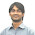Welcome! Dear Traders,you are reading my forex trading experiences. Forex trading is a very profitable and very risky business opportunity. If you are a beginner, calm down,have a cup of coffee, and convince yourself that you need to study hard to win in forex trading. Obviously, the task is not easy as the statistics claim that only 5% traders win in forex trading. If you are determined, serious,and hard working, you can surely be included in the group of winners.

HOW TO TRADE BEARISH AB=CD HARMONIC CHART PATTERN

Bearish AB=CD is a simple but widely found harmonic chart pattern drawn following some simple steps explained in this post.

Bearish AB=CD harmonic chart pattern suggests a short entry upon completion of the pattern. To trade the pattern, firstly traders need to find the pattern on the price chart.

How to find a bearish AB=CD harmonic chart pattern on price chart?

The fist step to find a Bearish AB=CD harmonic chart pattern is to find A and B points of the pattern. The A and B points are found at the bottom and top of a bullish trend respectively.

In the next step, we will find the C point of the bearish harmonic chart pattern. To find the B point, we will draw a fibonacci retracement tool from A to B. The B point should be at the 38.2-88.6% fibonacci retracement level of AB. Look at the following illustration.

In this step, we will draw a fibonacci retracement tool from B to C to confirm the D point of the bearish AB=CD harmonic chart pattern. The D point should be at the 113-261.8% fibonacci retracement level of BC.

How to trade the bearish AB=CD harmonic chart pattern?
When the D point of the bearish AB=CD harmonic chart pattern is confirmed, a short entry is suggested. The stop loss for the order at point D should be placed above the upper fibonacci retracement level or other resistance level.

The profit target for the above order should be placed at the AC trend line. In some cases, AC trend line can be broken by the price then the profit target can be placed at the 50-88.6%  fibonacci retracement level of CD. Following image explains such a situation.

This post explains the basics to trade bearish AB=CD harmonic chart pattern. If you want to join the discussion with other traders, you can drop a comment bellow. You can also subscribe yourFXguide to receive updates.

Thank You & Good Luck !!!

1.When should we considered D point confirmed as it is a range from 113 to 261%?

2.Thank you for your nice question. I hope I will edit my post later to answer this question. Okay, we know it is a range but still we can predict the D point. If BC is relatively large, you can expect that the D would be at the 113%. But if the BC is relatively small the D may be at the 261% retracement of BC.In another way, if the C is at the 38.2% fibonacci retracement of AB, the D can be at the 2.618% fibonacci retracement of BC. And, if C is at the 88.6% fibonacci retracement of AB, the D can be at the 113% fibonacci retracement of BC.

3.Hi
I have been studying this for a while and my take is that using any projection just indicates an impulse wave measured projection results in AB=CD

quote scott carney
"AB=CD Reciprocal Ratios
In the AB=CD pattern, the alignment of Fibonacci ratios within the structure usually manifests
specific reciprocal relationships. The reciprocal ratio of the C point retracement of the AB leg
usually indicates which BC projection is utilized to define the Potential Reversal Zone (PRZ).
For instance, a 0.618 retracement at the C point typically will possess a 1.618 BC projection
that converges closest with the completion of the AB=CD. This reciprocal relationship within the
equivalent AB=CD pattern defines the best PRZ for this structure. The reciprocal ratios that
complement the AB=CD structure are as follows:
C Point Retracement BC Projection
0.382 .24 or 2.618
0.50 2.0
0.618 1.618
0.707 1.41
0.786 1.27
0.886 1.13
The reciprocal ratios help define the completion of the pattern. However, the most
important consideration to remember is that the BC projection should converge closely with
the completion of the AB=CD."

4.I think this is an informative post and it is very useful and knowledgeable. therefore, I would like to thank you for the efforts you have made in writing this article. cd duplication services

Drop a comment...